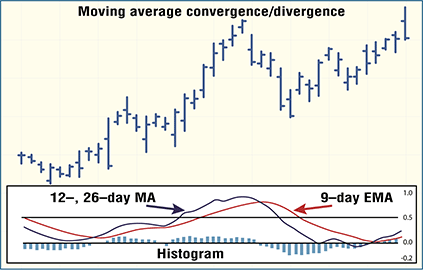### Indicators

#### 6. Moving Average Convergence/Divergence

A trend-following indicator devised by Gerald Appel in the 1970s, the moving average convergence/divergence (MACD) identifies crossovers, finds overbought/oversold conditions, and tracks divergences. Simply, the MACD is the crossing of two exponentially smoothed moving averages that are plotted above and below a zero line. The crossover, movement through the zero line, and divergences generate buy and sell signals.According to Steve Achelis, the MACD is most often calculated by subtracting a 26-day moving average of a tradable’s price from a 12-day moving average of its price, resulting in an indicator that oscillates above and below zero. Then the nine-day exponential moving average of the MACD line is plotted.

According to John J. Murphy, the MACD histogram is a variation, plotting the difference between the signal and MACD lines. The changes in the spread between the two lines can be spotted quicker, leading to earlier trading signals.

— EMS Flynn